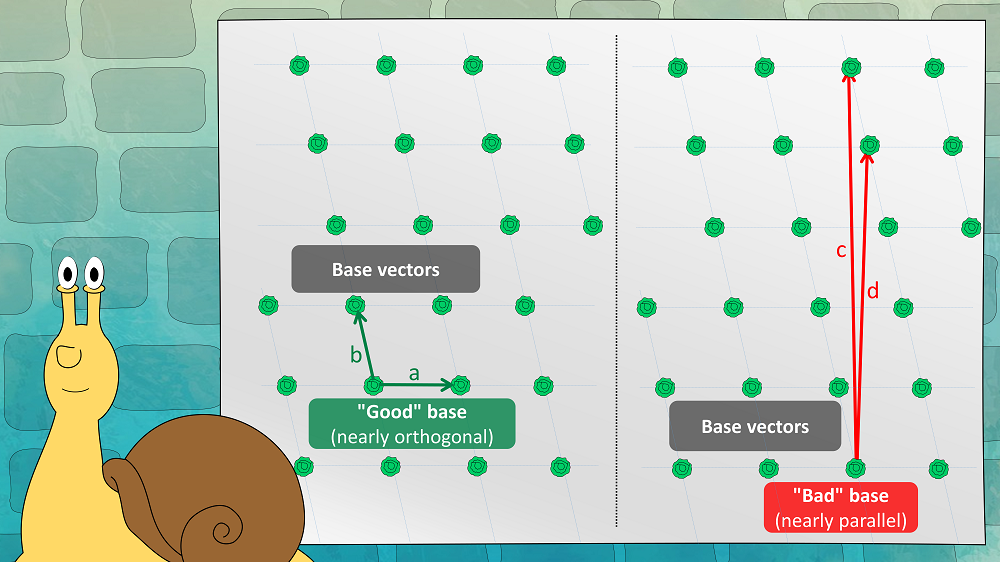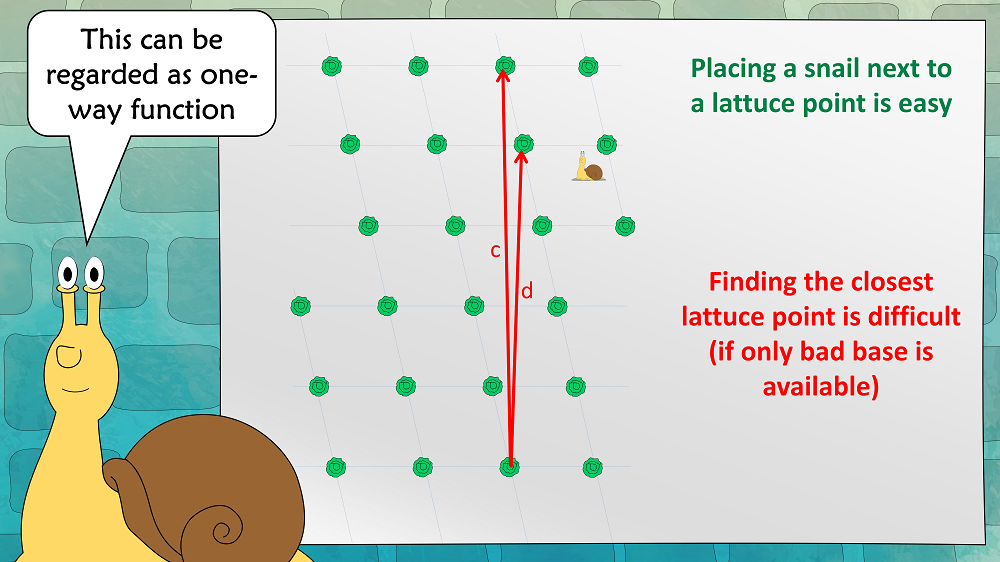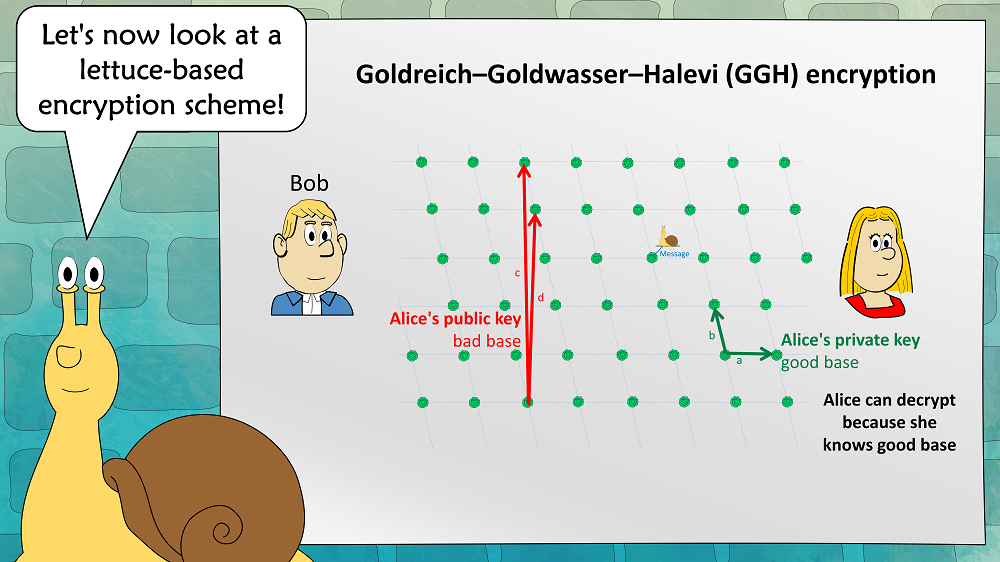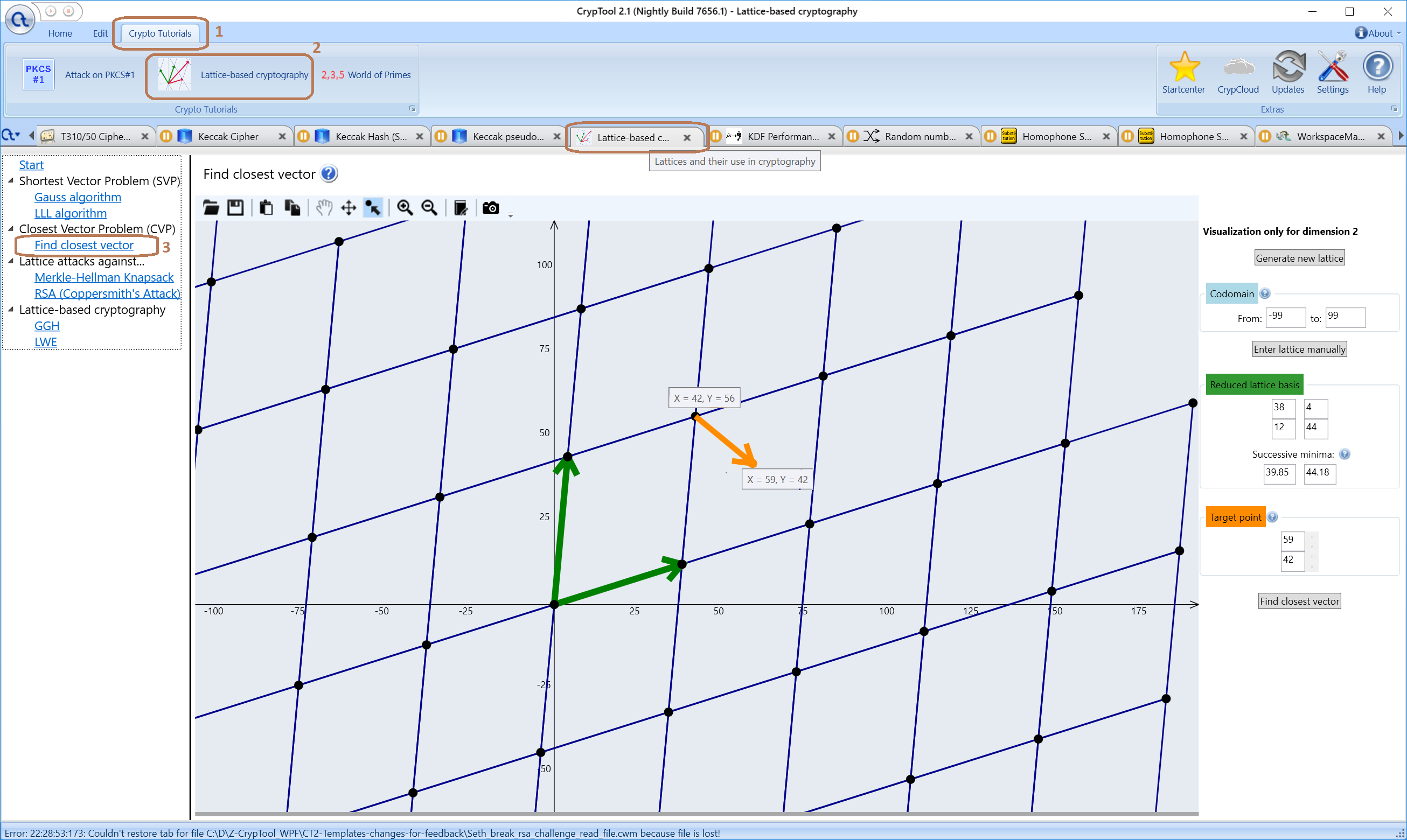# How I explained lettuce-based cryptography at a London conferenceAt the 44CON, a London-based hacker conference, I gave a talk about Post-Quantum cryptography. Among other things, I explained the GGH algorithm – using snail terminology.

How to Explain Post-Quantum Cryptography to a Middle School Student, was the title of a presentation I gave at the 44CON conference in London last Friday.

Post-quantum cryptography, one of the hottest topics in IT security today, addresses asymmetric crypto systems that are not prone to quantum computers. Virtually all asymmetric crypto systems currently in use (especially, Diffie-Hellman, RSA, DSA, and Elliptic Curve crypto systems) are not post-quantum. They will be useless, once advanced quantum computers will be available. Quantum computer technology has made considerable progress in recent years, with major organisations, like Google, NSA, and NASA, investing in it.

Post-quantum cryptography uses advanced mathematical concepts. Even if one knows the basics of current asymmetric cryptography (integer factorisation, discrete logarithms, …), Post-quantum algorithms are hard to understand. The goal of my presentation was to explain post-quantum cryptography in a way that is comprehensible for non-mathematicians.

The following video shows a short excerpt from my talk:

One of the post-quantum methods I explained is the so-called GGH algorithm (named for its inventors Goldreich, Goldwasser, and Halevi). GGH is a lattice-based crypto algorithm. But how can one explain lattice-based cryptography in an entertaining and easy-to-understand way? My co-worker Daniel Rocholz from cryptovision had a great idea: explain it from the point-of-view of a snail. The following presentation slides show how I put Daniel’s idea into practice.

First of all, a snail certainly prefers lettuce to lattice:This means that in our explanations we replace lattices with lettuce fields:A lettuce field is defined by a set of vectors. This set is referred to as a “base”; the vectors are named “base-vectors”. In the two-dimensional case, there are two base-vectors. As can be seen in the next picture, there are good bases (nearly orthogonal) and bad bases (nearly parallel) for the same lettuce field:We now assume that a snail is placed somewhere in the lettuce field. As a snail is very slow, it has an interest in finding the closest lettuce. This results in the “closest lettuce” problem:If we assume that we are in a high-dimensional lettuce field, this problem is easy to solve with a good base, but hard to solve with a bad base.

This problem can also be regarded as a one-way function:Now we can define the GGH asymmetric encryption scheme:Alices public key is a bad base, her private key is a good base. To encrypt, Bob places a snail near a lettuce. The vector between the snail and the lettuce is the cleartext (in practice, the lettuce field is about 250-dimensional, which means that this vector can easily encode a 128 bit message). Alice can easily find the closest lettuce (and thus decrypt the message), as she knows a good base. An attacker can’t, as he has only a bad base.

That’s it. This isn’t very difficult, is it?

Unfortunately, GGH has proven insecure, so it should not be used in practice. However, there are other lattice-/lettuce-based encryption schemes, that are considered secure, like NTRU, (R)LWE, and New Hope. 27 algorithms of this kind have been handed in to the NIST Post-Quantum Competition. I’m pretty sure that several of them will be among the winners.

## Kommentare (7)

1. #1 Klaus Schmeh18. September 2018

Well I felt like a vegetable just trying to understand even the snail version. Maybe I’ll try again tomato… I mean, tomorrow.

2. #2 Bernhard Esslinger18. September 2018

I had to laugh — this is a great and creative idea. The pupils will
keep the snail and the lettuces in their mind and so they got the idea.
Older pupils will turn this to their vector analysis with the difference
that the coefficients of the two vectors are only integers so by adding
them you don’t span the whole plane as vector space, but only the lattice.

In CrypTool 2 there is a tutorial about lattice crypto which shows the
ideas of the easiest problems and methods in 2 dimensions graphically.
Attached is a screenshot for the closest vector problem: Click “Crypto
tutorials”, then “Lattice-based cryptography”, and then “Find closest
vector”. The 2 green vectors span the lattice. The orange point [here
(59/42)] is set manually and arbitrarily. The orange vector shows the
way to the closest lattice point (42/56).You can play around to get the ideas, and zoom in and out, set new
orange points or new green base vectors. Just click to the icon “Set
target point”, click around in the vector space, and you will see the
closest lattice at once.

3. #3 Сhemіkеr19. September 2018

If I have understood the method correctly, then it relies on a private key (which is a good base) in which the solution is easy to find, while the public key (a bad base) just allows you to ask the problem and check the result, but finding the nearest lattice point is a hard problem.

On the other hand, orthonalizing a given base is not really difficult, even in a few hundred dimensions. What prevents an attacker from converting a bad into a good base?

4. #4 Klaus Schmeh19. September 2018

@Chemiker:
>orthonalizing a given base is not really difficult, even
>in a few hundred dimensions. What prevents an
>attacker from converting a bad into a good base?
As far as I know, deriving a good base from a bad one is difficult (at least, it is extremely laborious). Otherwise, this scheme would not work.

5. #5 BE19. September 2018

It’s difficult in the discrete space in contrast to the continuous space.

6. #6 Сhemіkеr20. September 2018

@BE Thanks, the ‘discrete’ information was missing (at least, for me). Whatever a ‘discrete’ basis is.

7. #7 Joshua Holdenhttp://www.rose-hulman.edu/~holden
28. September 2018

The usual way of orthogonalizing a basis (Gram-Schmidt) will result in rational numbers in the basis. So they will fall in between the lattice points. A discrete basis in this situation means it has to stick to the lattice points.